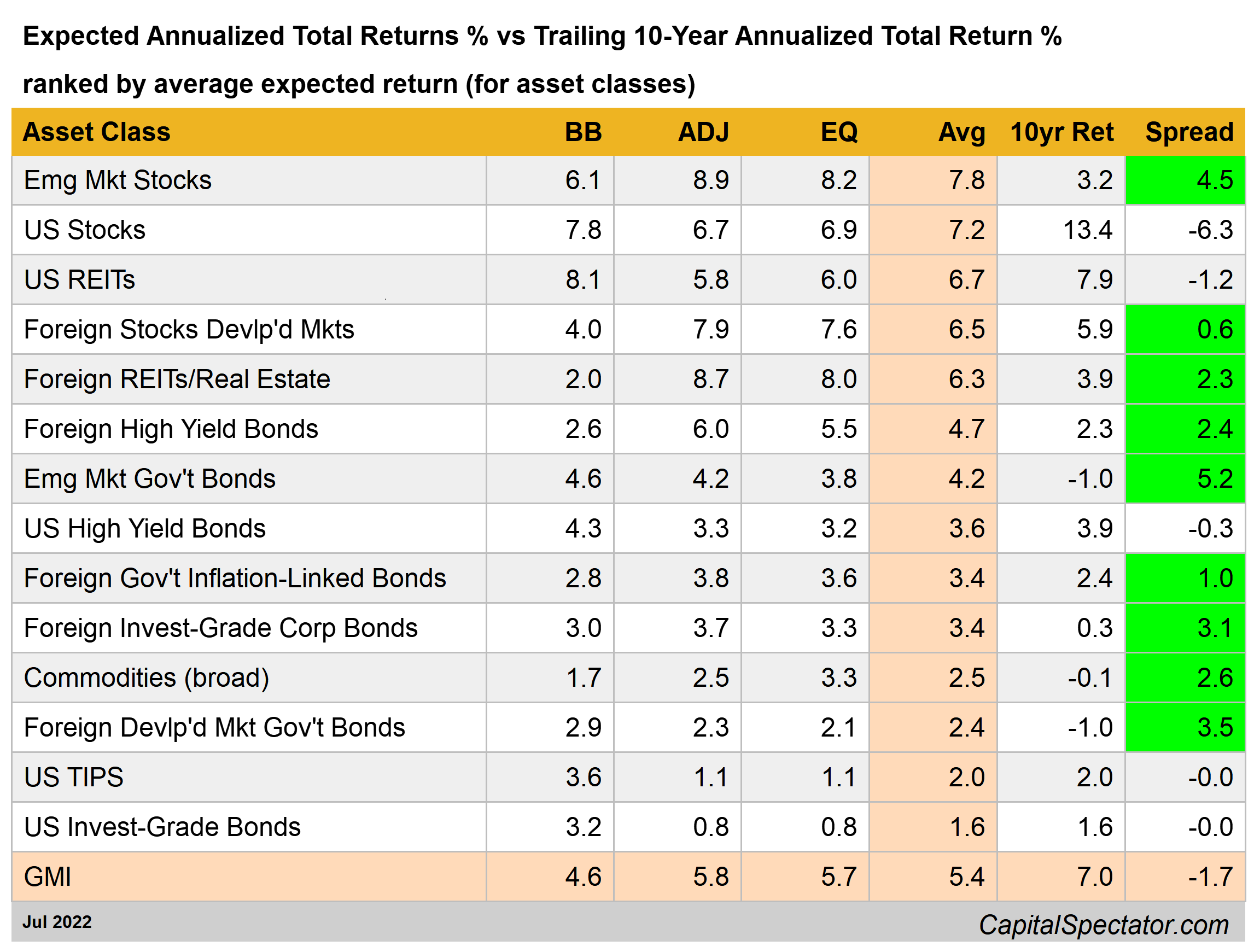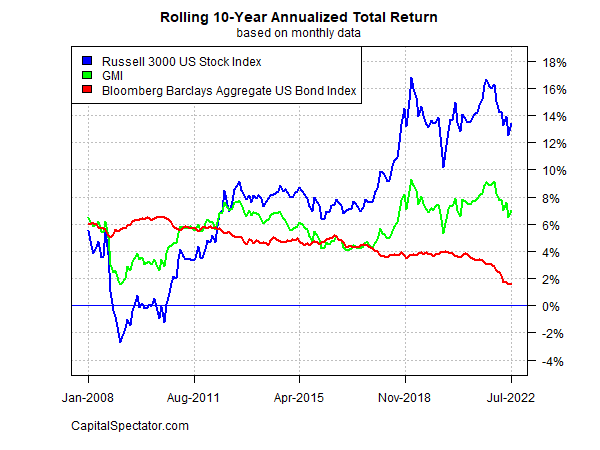# Total Return Forecasts: Major Asset Classes | 2 August 2022

The long-term expected return for the Global Market Index (GMI) remains moderately below the index’s trailing realized 10-year performance through July, based on the average of three forecasting models (defined below). The key takeaway: it’s still prudent to manage expectations down for multi-asset-class strategies, relative to the past decade.

The table below offers a first approximation for developing long-run return estimates for the major asset classes and GMI, an unmanaged, market-value-weighted portfolio that holds all the major asset classes (except cash) and represents a theoretical benchmark of the optimal portfolio for the average investor with an infinite time horizon. On that basis, GMI is useful as a starting point for research on asset allocation and portfolio design. GMI’s history suggests that this passive benchmark’s performance is competitive with most active asset-allocation strategies overall, especially after adjusting for risk, trading costs and taxes.Returns highlighted in green represent relatively attractive return forecasts. These are asset classes with average return expectations above the relevant trailing 10-year performance.

Keep in mind that all forecasts will likely be incorrect in some degree, although GMI’s projections are expected to be more reliable vs. the estimates for the individual asset classes shown in the table above. Predictions for the specific market components (US stocks, commodities, etc.) are subject to greater volatility and tracking error compared with aggregating forecasts into the GMI estimate, a process that may reduce some of the errors through time.

For historical perspective on how GMI’s realized total return has evolved through time, consider the benchmark’s history on a rolling 10-year annualized basis. The chart below compares GMI’s performance vs. the equivalent for US stocks (Russell 3000) and US Bonds (Bloomberg Aggregate Bond) through last month. GMI’s current 10-year return (green line) is a solid 7.0%. While that’s fallen from recent levels, it’s still well above the current long-run projection. That’s an indication to remain relatively cautious for projecting returns for multi-asset-class portfolios vs. the historical record over the past decade.Here’s a brief summary of how the forecasts are generated:

BB: The Building Block model uses historical returns as a proxy for estimating the future. The sample period used starts in January 1998 (the earliest available date for all the asset classes listed above). The procedure is to calculate the risk premium for each asset class, compute the annualized return and then add an expected risk-free rate to generate a total return forecast. For the expected risk-free rate, we’re using the latest yield on the 10-year Treasury Inflation Protected Security (TIPS). This yield is considered a market estimate of a risk-free, real (inflation-adjusted) return for a “safe” asset — this “risk-free” rate is also used for all the models outlined below. Note that the BB model used here is (loosely) based on a methodology originally outlined by Ibbotson Associates (a division of Morningstar).

EQ: The Equilibrium model reverse engineers expected return by way of risk. Rather than trying to predict return directly, this model relies on the somewhat more reliable framework of using risk metrics to estimate future performance. The process is relatively robust in the sense that forecasting risk is slightly easier than projecting return. The three inputs:

* An estimate of the overall portfolio’s expected market price of risk, defined as the Sharpe ratio, which is the ratio of risk premia to volatility (standard deviation). Note: the “portfolio” here and throughout is defined as GMI

* The expected volatility (standard deviation) of each asset (GMI’s market components)

* The expected correlation for each asset relative to the portfolio (GMI)

This model for estimating equilibrium returns was initially outlined in a 1974 paper by Professor Bill Sharpe. For a summary, see Gary Brinson’s explanation in Chapter 3 of The Portable MBA in Investment. I also review the model in my book Dynamic Asset Allocation. Note that this methodology initially estimates a risk premium and then adds an expected risk-free rate to arrive at total return forecasts. The expected risk-free rate is outlined in BB above.

ADJ: This methodology is identical to the Equilibrium model (EQ) outlined above with one exception: the forecasts are adjusted based on short-term momentum and longer-term mean reversion factors. Momentum is defined as the current price relative to the trailing 12-month moving average. The mean reversion factor is estimated as the current price relative to the trailing 60-month (5-year) moving average. The equilibrium forecasts are adjusted based on current prices relative to the 12-month and 60-month moving averages. If current prices are above (below) the moving averages, the unadjusted risk premia estimates are decreased (increased). The formula for adjustment is simply taking the inverse of the average of the current price to the two moving averages. For example: if an asset class’s current price is 10% above its 12-month moving average and 20% over its 60-month moving average, the unadjusted forecast is reduced by 15% (the average of 10% and 20%). The logic here is that when prices are relatively high vs. recent history, the equilibrium forecasts are reduced. On the flip side, when prices are relatively low vs. recent history, the equilibrium forecasts are increased.

Avg: This column is a simple average of the three forecasts for each row (asset class)

10yr Ret: For perspective on actual returns, this column shows the trailing 10-year annualized total return for the asset classes through the current target month.

Spread: Average-model forecast less trailing 10-year return.

Learn To Use R For Portfolio Analysis
Quantitative Investment Portfolio Analytics In R:
An Introduction To R For Modeling Portfolio Risk and ReturnBy James Picerno

This site uses Akismet to reduce spam. Learn how your comment data is processed.